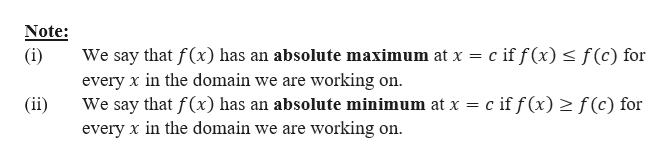# Find the absolute maximum and minimum values of the following function on the given interval. Then graph the function9Pf(x) -x9, -4sxs3Find the absolute maximum value. Select the correct choice below and, if necessary, fill in the answer boxes to complete your choice.A. The absolute maximum valueOCcurs at x =(Simplify your answers. Use a comma to separate answers as needed.)B. There is no absolute maximum.Find the absolute minimum value. Select the correct choice below and, if necessary, fill in the answer boxes to complete your choice.O A. The absolute minimum valueOCcurs at x =(Simplify your answers. Use a comma to separate answers as needed.)B. There is no absolute minimumChoose the correct graph of the function.O C.O D.O A.O B.Ay10-10-10-10-חnתהר-1010-1010-1010-1010-10-10-10-10

Question
33 views

Can you help me step by step?

check_circle

Step 1

Here we are given that f(x) = -x2 + 9, {-4 ≤ x ≤ 3}. We need to find the absolute maximum and minimum values of the above function on the given interval.help_outlineImage TranscriptioncloseNote: We say that f(x) has an absolute maximum at x c if f (x) s f(c) for (i) every x in the domain we are working on (ii) cif f(x) 2 f(c) for We say that f(x) has an absolute minimum at x every x in the domain we are working on. fullscreen
Step 2

If we draw the graph, we get, Absolute maxima...

### Want to see the full answer?

See Solution

#### Want to see this answer and more?

Solutions are written by subject experts who are available 24/7. Questions are typically answered within 1 hour.*

See Solution
*Response times may vary by subject and question.
Tagged in

### Calculus